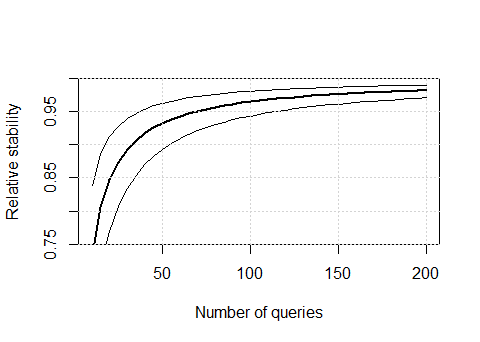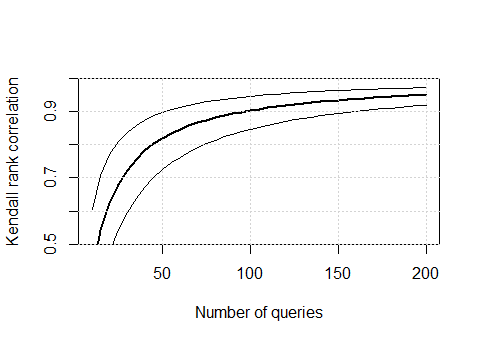# gt4ireval: Generalizability Theory for Information Retrieval Evaluation

#### 2017-03-06

gt4ireval is a package to measure the reliability of an Information Retrieval test collection. It allows users to estimate reliability using Generalizability Theory (Brennan 2001) and map those estimates onto well-known indicators such as Kendall $$\tau$$ correlation or sensitivity. For background information and details, the reader is referred to (Urbano, Marrero, and Martín 2013).

Once loaded, gt4ireval needs initial evaluation data to run a G-study and the corresponding D-study. These data need to be in a standard data frame or matrix, where columns correspond to systems and rows correspond to queries.1 For this vignette, let us use data from the TREC-3 Ad hoc track.

dim(adhoc3)
##  50 40
adhoc3[1:5, 1:5]
##     sys1   sys2   sys3   sys4   sys5
## 1 0.2830 0.5163 0.4810 0.5737 0.5184
## 2 0.0168 0.5442 0.3987 0.2964 0.6115
## 3 0.0746 0.2769 0.3002 0.2459 0.3803
## 4 0.1828 0.6622 0.6164 0.4291 0.6556
## 5 0.0181 0.3670 0.3762 0.1095 0.2465

If your data is transposed (i.e. columns correspond to queries and rows correspond to systems), you can get the correct format with the t function: data <- t(data).

## G-Study

To run a G-study with the initial data we have, we simply call function g.study.

gstudy(adhoc3)
##
## Summary of G-Study
##
##                  Systems     Queries Interaction
##              ----------- ----------- -----------
## Variance       0.0071668    0.022642     0.01092
## Variance(%)       17.596      55.593      26.811
## ---
## Mean Sq.         0.36926     0.91661     0.01092
## Sample size           40          50        2000

Additionally, we can tell the function to ignore the systems with lowest average effectiveness scores by setting parameter drop. For instance, we can ignore the bottom 25% of systems.

adhoc3.g <- gstudy(adhoc3, drop = .25)
adhoc3.g
##
## Summary of G-Study
##
##                  Systems     Queries Interaction
##              ----------- ----------- -----------
## Variance       0.0028117    0.028093    0.010152
## Variance(%)       6.8482      68.425      24.727
## ---
## Mean Sq.         0.15074     0.85296    0.010152
## Sample size           30          50        1500

The summary shows the estimated variance components: variance due to the system effect $$\hat\sigma_s^2=0.0028$$, due to the query effect $$\hat\sigma_q^2=0.0281$$, and due to the system-query interaction effect $$\hat\sigma_e^2=0.0102$$. The second row shows the same values but as a fraction of the total variance. The third row shows the estimated Mean Squares for each component, and finally the sample size in each case. In our example, we have 30 systems and 50 queries as initial data.

## D-Study

The results from the G-study above can now be used to run a D-study. First, let us estimate the stability of the current collection (50 queries).

dstudy(adhoc3.g)
##
## Summary of D-Study
##
## Call:
##     queries = 50
##   stability = 0.95
##       alpha = 0.025
##
## Stability:
##                                            Erho2                                   Phi
##              -----------------------------------   -----------------------------------
##      Queries    Expected       Lower       Upper      Expected       Lower       Upper
##  ----------- ----------- ----------- -----------   ----------- ----------- -----------
##           50     0.93265     0.89311     0.96287       0.78613     0.66141     0.88039
##
## Required number of queries:
##                                            Erho2                                   Phi
##              -----------------------------------   -----------------------------------
##    Stability    Expected       Lower       Upper      Expected       Lower       Upper
##  ----------- ----------- ----------- -----------   ----------- ----------- -----------
##         0.95          69          37         114           259         130         487

The summary first shows how dstudy was called. In particular, it tells us that the target number of queries is $$n_q'=50$$ (set by default from the G-study initial data), the target stability is $$\pi=0.95$$ (set by default), and the confidence level is $$\alpha=0.025$$ (set by default). Next are the estimated stability scores; the relative stability with 50 queries is $$\text{E}\hat\rho^2=0.93265$$ with a 95% confidence interval of $$[0.89311, 0.96287]$$, and the absolute stability is $$\hat\Phi=0.78613$$ with a 95% confidence interval of $$[0.66141, 0.88039]$$. Regarding the required number of queries to reach the target stability, the estimate is $$\hat{n}_q'=69$$ with a 95% confidence interval of $$[37, 114]$$ to reach $$\text{E}\rho^2=\pi$$, and $$\hat{n}_q'=259$$ with a 95% confidence interval of $$[130, 487]$$ to reach $$\Phi=\pi$$.

Function dstudy can be called with multiple values for $$n_q'$$, $$\pi$$ and $$\alpha$$ to study trends. For instance, we can indicate several query set sizes by setting parameter queries.

dstudy(adhoc3.g, queries = seq(20, 200, 20))
##
## Summary of D-Study
##
## Call:
##     queries = 20 40 60 80 100 120 140 160 180 200
##   stability = 0.95
##       alpha = 0.025
##
## Stability:
##                                            Erho2                                   Phi
##              -----------------------------------   -----------------------------------
##      Queries    Expected       Lower       Upper      Expected       Lower       Upper
##  ----------- ----------- ----------- -----------   ----------- ----------- -----------
##           20     0.84707     0.76971     0.91208        0.5952     0.43864     0.74647
##           40     0.91721     0.86987     0.95402       0.74624      0.6098     0.85483
##           60     0.94324     0.90931     0.96887       0.81519     0.70097      0.8983
##           80     0.95682     0.93041     0.97647       0.85468     0.75761     0.92174
##          100     0.96515     0.94354     0.98109       0.88026     0.79621     0.93639
##          120     0.97079      0.9525     0.98419       0.89819      0.8242     0.94643
##          140     0.97486     0.95901     0.98642       0.91144     0.84543     0.95373
##          160     0.97793     0.96395     0.98809       0.92165     0.86209     0.95927
##          180     0.98033     0.96783      0.9894       0.92974      0.8755     0.96363
##          200     0.98227     0.97095     0.99045       0.93632     0.88654     0.96715
##
## Required number of queries:
##                                            Erho2                                   Phi
##              -----------------------------------   -----------------------------------
##    Stability    Expected       Lower       Upper      Expected       Lower       Upper
##  ----------- ----------- ----------- -----------   ----------- ----------- -----------
##         0.95          69          37         114           259         130         487

The output above shows the estimated stability scores, with confidence intervals, for various query set sizes. For example, we have $$\text{E}\hat\rho^2=0.96515$$ with 100 queries, and $$\hat\Phi\in[0.88654, 0.96715]$$ with 95% confidence when having 200 queries. Similarly, we may indicate several target stability scores by setting parameter stability.

dstudy(adhoc3.g, stability = c(0.8, 0.85, 0.9, 0.95, 0.97, 0.99))
##
## Summary of D-Study
##
## Call:
##     queries = 50
##   stability = 0.8 0.85 0.9 0.95 0.97 0.99
##       alpha = 0.025
##
## Stability:
##                                            Erho2                                   Phi
##              -----------------------------------   -----------------------------------
##      Queries    Expected       Lower       Upper      Expected       Lower       Upper
##  ----------- ----------- ----------- -----------   ----------- ----------- -----------
##           50     0.93265     0.89311     0.96287       0.78613     0.66141     0.88039
##
## Required number of queries:
##                                            Erho2                                   Phi
##              -----------------------------------   -----------------------------------
##    Stability    Expected       Lower       Upper      Expected       Lower       Upper
##  ----------- ----------- ----------- -----------   ----------- ----------- -----------
##          0.8          15           8          24            55          28         103
##         0.85          21          11          34            78          39         146
##          0.9          33          18          54           123          62         231
##         0.95          69          37         114           259         130         487
##         0.97         117          63         194           440         220         828
##         0.99         358         191         593          1347         673        2534

The output above shows that the estimated number of queries to reach $$\text{E}\rho^2=0.97$$ is 117, while 123 are required to reach $$\Phi=0.9$$. Finally, we can also indicate several confidence levels for the computation of confidence intervals by setting parameter alpha.2

dstudy(adhoc3.g, alpha = c(0.005, 0.025, 0.05))
##
## Summary of D-Study
##
## Call:
##     queries = 50
##   stability = 0.95
##       alpha = 0.005 0.025 0.05
##
## Stability:
##                                            Erho2                                   Phi
##              -----------------------------------   -----------------------------------
##        Alpha    Expected       Lower       Upper      Expected       Lower       Upper
##  ----------- ----------- ----------- -----------   ----------- ----------- -----------
##        0.005     0.93265     0.87737     0.96967       0.78613     0.61466      0.9023
##        0.025     0.93265     0.89311     0.96287       0.78613     0.66141     0.88039
##         0.05     0.93265     0.90062     0.95901       0.78613     0.68417     0.86796
##
## Required number of queries:
##                                            Erho2                                   Phi
##              -----------------------------------   -----------------------------------
##        Alpha    Expected       Lower       Upper      Expected       Lower       Upper
##  ----------- ----------- ----------- -----------   ----------- ----------- -----------
##        0.005          69          30         133           259         103         596
##        0.025          69          37         114           259         130         487
##         0.05          69          41         105           259         145         439

The summary above shows that with 50 queries a 99% confidence interval for $$\text{E}\rho^2$$ is $$[0.87737, 0.96967]$$, and a 90% confidence interval on the number of queries to reach $$\Phi=0.95$$ is $$[145, 439]$$.

## Using the Returned Objects

Both gstudy and dstudy return objects with all results from the analysis so they can be used in subsequent computations. In fact, object adhoc3.g above contains all the G-study results, and it is provided to function d.study. The full list of available data in both objects can be obtained with function names.

adhoc3.g <- gstudy(adhoc3, drop = 0.25)
names(adhoc3.g)
##  "n.s"   "n.q"   "var.s" "var.q" "var.e" "em.s"  "em.q"  "em.e"  "call"
adhoc3.g$var.s ##  0.002811699 adhoc3.d <- dstudy(adhoc3.g, queries = seq(10, 100, 10), stability = seq(0.5, 0.99, .05)) names(adhoc3.d) ##  "Erho2" "Phi" "n.q_Erho2" "n.q_Phi" ##  "Erho2.lwr" "Erho2.upr" "Phi.lwr" "Phi.upr" ##  "n.q_Erho2.lwr" "n.q_Erho2.upr" "n.q_Phi.lwr" "n.q_Phi.upr" ##  "call" adhoc3.d$Erho2
##   0.7347152 0.8470730 0.8925725 0.9172057 0.9326493 0.9432373 0.9509485
##   0.9568151 0.9614284 0.9651511
cbind(lwr = adhoc3.d$n.q_Phi.lwr, upr = adhoc3.d$n.q_Phi.upr)
##       lwr upr
##  [1,]  26   7
##  [2,]  32   9
##  [3,]  39  11
##  [4,]  48  13
##  [5,]  60  16
##  [6,]  77  21
##  [7,] 103  28
##  [8,] 146  39
##  [9,] 231  62
## [10,] 487 130

With all these data we can for instance plot the estimated $$\text{E}\hat\rho^2$$ score, with a 95% confidence interval, as a function of the number of queries in the collection.

xx <- seq(10, 200, 5)
plot(xx, adhoc3.d$Erho2, yaxs = "i", ylim = c(0.75, 1), lwd = 2, type = "l", xlab = "Number of queries", ylab = "Relative stability") lines(xx, adhoc3.d$Erho2.lwr) # lower confidence limit
lines(xx, adhoc3.d$Erho2.upr) # upper confidence limit grid()## Mapping G-Theory onto Data-based Indicators Finally, the following functions can be used to map stability indicators from Generalizability Theory onto well-known data-based indicators (see (Urbano, Marrero, and Martín 2013) for details): • gt2tau and gt2tauAP map $$\text{E}\rho^2$$ onto Kendall $$\tau$$ correlation and $$AP$$ correlation coefficients. • gt2power, gt2minor and gt2major map $$\text{E}\rho^2$$ onto expected power, minor conflict rate and major conflict rate of 2-tailed t-tests. • gt2asens and gt2rsens map $$\text{E}\rho^2$$ and $$\Phi$$ onto absolute and relative sensitivity, respectively. • gt2rmse maps $$\Phi$$ onto rooted mean squared error. gt2tau(Erho2 = 0.95) ##  0.8641168 gt2rsens(Phi = 0.8) ##  0.1238861 The results show that the estimated rank correlation at $$\text{E}\rho^2=0.95$$ is $$\hat\tau=0.86412$$, and that the relative sensitivity at $$\Phi=0.8$$ is estimated as $$\hat\delta_r=12.389\%$$. In order to map the stability of a certain D-study, we can simply use the returned dstudy object. These functions can be used for instance to plot the estimated $$\hat\tau$$ correlation as a function of the query set size. xx <- seq(10, 200, 5) adhoc3.d <- dstudy(adhoc3.g, queries = xx) plot(xx, gt2tau(adhoc3.d$Erho2),
yaxs = "i", ylim = c(0.5, 1), lwd = 2, type = "l",
xlab = "Number of queries", ylab = "Kendall rank correlation")
lines(xx, gt2tau(adhoc3.d$Erho2.lwr)) # lower confidence limit lines(xx, gt2tau(adhoc3.d$Erho2.upr)) # upper confidence limit
grid()In any case, the user is strongly advised to take these mappings with a grain of salt (see Fig. 3 in (Urbano, Marrero, and Martín 2013)).

### Acknowledgements

This work was supported by an A4U postdoctoral grant and a Juan de la Cierva postdoctoral fellowship.

1. For general information on how to read data in R, the reader is referred to the R Data Import/Export manual.

2. Recall that $$100(1-2\alpha)\%$$ intervals are computed, so for an 80% confidence interval we set $$\alpha=0.1$$.